# Test: Comparing Quantities- 1

## 10 Questions MCQ Test Mathematics (Maths) Class 8 | Test: Comparing Quantities- 1

Description
Attempt Test: Comparing Quantities- 1 | 10 questions in 10 minutes | Mock test for Class 8 preparation | Free important questions MCQ to study Mathematics (Maths) Class 8 for Class 8 Exam | Download free PDF with solutions
QUESTION: 1

### Find the ratio of speed of a cycle 15 km per hour to the speed of scooter 30 km per hour.

Solution:

Speed of cycle = 15 km per hour

Speed of scooter = 30 km per hour

∴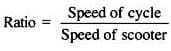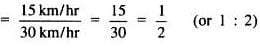QUESTION: 2

### Rohan bought a second hand refrigerator for Rs 2,500, then spent Rs 500 on its repairs and sold it for Rs 3,300. Find his loss or gain per cent.

Solution:

Cost of refrigerator = Rs 2500

Money spent on repairs = Rs 500

Total CP of refrigerator = Rs 2500 + 500 = Rs 3000

SP of refrigerator = Rs 3300

Since SP > CP, so profit ocurrs.

Now, profit = SP − CP = 3300 − 3000 = Rs 300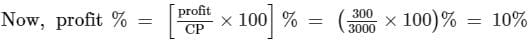QUESTION: 3

### A picnic is being planned in a school for Class VII. Girls are 60% of the total number of students and are 18 in number. Find the ratio of the number of girls to the number of boys in the class.

Solution:

Girls are 60% of the total number of students and are 18 in number.

Let say total students = T

Girls = (60/100)T

=> 18 = 3T/5

=> T = 30

Boys = 30 - 18  = 12

Ratio of Girls to boys = 18 : 12

=> 3 : 2

QUESTION: 4

During a sale, a shop offered a discount of 10% on the marked prices of all the items. What would a customer have to pay for a pair of jeans marked at Rs 1450 and two shirts marked at Rs 850 each?

Solution: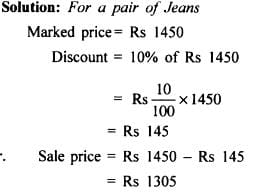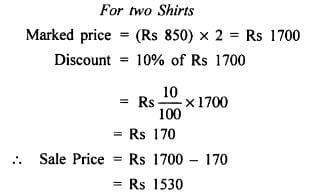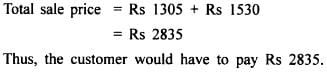QUESTION: 5

Calculate compound interest on Rs 10,800 for 3 years at 12.5% per annum compounded annually.

Solution: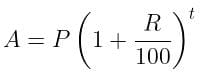P = Rs. 10800
R = 12.5%
t = 3 years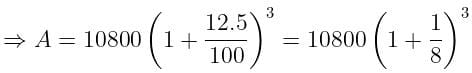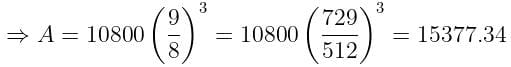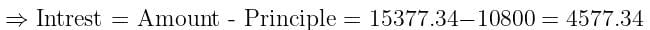QUESTION: 6

A sum of money, at compound interest, yields Rs. 200 and Rs. 220 at the end of first and second years respectively. What is the rate percent?

Solution:

Difference between interests = Rs.220 - Rs. 200 = Rs. 20
⇒ Rs. 20 is the S.I on Rs. 200 for 1 Year.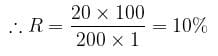Hence, the rate of interest is 10%.

QUESTION: 7

The population of a city was 20,000 in the year 1997. It increased at the rate of 5% p.a. Find the population at the end of the year 2000.

Solution:

We have,
P = 20,000, R = 5%, T = 3 year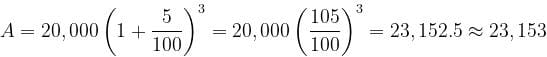QUESTION: 8

A shopkeeper purchased 200 bulbs for Rs 10 each. However 5 bulbs were fused and had to be thrown away. The remaining were sold at Rs 12 each. Find the gain or loss %.

Solution: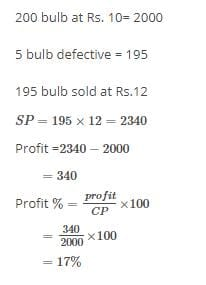QUESTION: 9

________ means comparing two quantities.

Solution:

A ratio is a relationship between two numbers indicating how many times the first number contains the second.
Example: If a bowl of fruit contains eight oranges and six lemons, then the ratio of oranges to lemons = 8:6 = 4:3

QUESTION: 10

The price of a scooter was Rs 34,000 last year. It has increased by 20% this year. What is the price now?

Solution: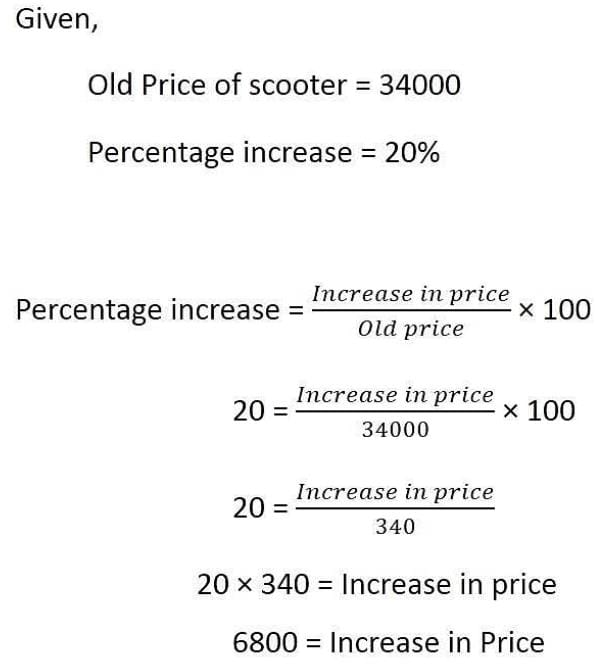Thus, Present price is Rs 40,800.Use Code STAYHOME200 and get INR 200 additional OFF Use Coupon Code